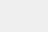# 知识图谱:一种从文本中挖掘信息的强大数据科学技术### 概览

• 知识图谱是数据科学中最有趣的概念之一

• 了解如何使用Wikipedia页面上的文本构建知识图谱

• 我们将动手使用Python流行的spaCy库构建我们的知识图谱

### 什么是知识图谱？### 句子分割

“Indian tennis player Sumit Nagal moved up six places from 135 to a career-best 129 in the latest men’s singles ranking. The 22-year-old recently won the ATP Challenger tournament. He made his Grand Slam debut against Federer in the 2019 US Open. Nagal won the first set.”

1. Indian tennis player Sumit Nagal moved up six places from 135 to a career-best 129 in the latest men’s singles ranking

2. The 22-year-old recently won the ATP Challenger tournament

3. He made his Grand Slam debut against Federer in the 2019 US Open

4. Nagal won the first set

### 实体提取

``import spacynlp = spacy.load('en_core_web_sm')doc = nlp("The 22-year-old recently won ATP Challenger tournament.")for tok in doc:  print(tok.text, "...", tok.dep_)``

Output:

``The … det22-year … amod– … punctold … nsubjrecently … advmodwon … ROOTATP … compoundChallenger … compoundtournament … dobj. … punct``

“22-year”的依赖项标签是amod，这意味着它是“old”的修饰语。因此，我们应该定义一个规则来提取这些实体。

### 提取关系

``doc = nlp("Nagal won the first set.")for tok in doc:  print(tok.text, "...", tok.dep_)``

Output:

``Nagal … nsubjwon … ROOTthe … detfirst … amodset … dobj. … punct``

### 根据文本数据构建知识图谱

#### 导入库

``import reimport pandas as pdimport bs4import requestsimport spacyfrom spacy import displacynlp = spacy.load('en_core_web_sm')from spacy.matcher import Matcher from spacy.tokens import Span import networkx as nximport matplotlib.pyplot as pltfrom tqdm import tqdmpd.set_option('display.max_colwidth', 200)%matplotlib inline``

#### 读取数据

``# 读取wikipedia句子candidate_sentences = pd.read_csv("wiki_sentences_v2.csv")candidate_sentences.shape``

Output:

``(4318, 1)``

``candidate_sentences['sentence'].sample(5)``

Output:

``doc = nlp("the drawdown process is governed by astm standard d823")for tok in doc:  print(tok.text, "...", tok.dep_)``

Output:

### 实体对提取

``def get_entities(sent):  ## chunk 1  ent1 = ""  ent2 = ""  prv_tok_dep = ""    # 句子中先前标记的依赖项标签  prv_tok_text = ""   # 句子中的前一个标记  prefix = ""  modifier = ""  #############################################################  for tok in nlp(sent):    ## chunk 2    # 如果标记是标点符号，则继续下一个标记    if tok.dep_ != "punct":      # 检查：标记是否为compound      if tok.dep_ == "compound":        prefix = tok.text        # 如果前一个单词也是'compound'，然后将当前单词添加到其中        if prv_tok_dep == "compound":          prefix = prv_tok_text + " "+ tok.text      # 检查：标记是否为修饰符      if tok.dep_.endswith("mod") == True:        modifier = tok.text        # 如果前一个单词也是'compound'，然后将当前单词添加到其中        if prv_tok_dep == "compound":          modifier = prv_tok_text + " "+ tok.text      ## chunk 3      if tok.dep_.find("subj") == True:        ent1 = modifier +" "+ prefix + " "+ tok.text        prefix = ""        modifier = ""        prv_tok_dep = ""        prv_tok_text = ""            ## chunk 4      if tok.dep_.find("obj") == True:        ent2 = modifier +" "+ prefix +" "+ tok.text      ## chunk 5        # 更新变量      prv_tok_dep = tok.dep_      prv_tok_text = tok.text  #############################################################  return [ent1.strip(), ent2.strip()]``

chunk1:

chunk 2:

chunk 3:

chunk 4:

chunk 5:

``get_entities("the film had 200 patents")``

Output:

``[‘film’, ‘200 patents’]``

Output:

### 关系/谓词提取

``def get_relation(sent):  doc = nlp(sent)  # Matcher类对象  matcher = Matcher(nlp.vocab)  #定义模式   pattern = [{'DEP':'ROOT'},             {'DEP':'prep','OP':"?"},            {'DEP':'agent','OP':"?"},              {'POS':'ADJ','OP':"?"}]   matcher.add("matching_1", None, pattern)   matches = matcher(doc)  k = len(matches) - 1  span = doc[matches[k]:matches[k]]   return(span.text)``

``  get_relation("John completed the task")``

Output:

``completed``

``relations = [get_relation(i) for i in tqdm(candidate_sentences['sentence'])]``

``pd.Series(relations).value_counts()[:50]``

Output:

### 建立知识图谱

``# 抽取主语source = [i for i in entity_pairs]# 抽取宾语target = [i for i in entity_pairs]kg_df = pd.DataFrame({'source':source, 'target':target, 'edge':relations})``

``# 从一个dataframe中创建一个有向图G=nx.from_pandas_edgelist(kg_df, "source", "target",                           edge_attr=True, create_using=nx.MultiDiGraph())``

``plt.figure(figsize=(12,12))pos = nx.spring_layout(G)nx.draw(G, with_labels=True, node_color='skyblue', edge_cmap=plt.cm.Blues, pos = pos)plt.show()``

Output:

``G=nx.from_pandas_edgelist(kg_df[kg_df['edge']=="composed by"], "source", "target",                           edge_attr=True, create_using=nx.MultiDiGraph())plt.figure(figsize=(12,12))pos = nx.spring_layout(G, k = 0.5) # k调节节点之间的距离nx.draw(G, with_labels=True, node_color='skyblue', node_size=1500, edge_cmap=plt.cm.Blues, pos = pos)plt.show()``

Output:``G=nx.from_pandas_edgelist(kg_df[kg_df['edge']=="written by"], "source", "target",                           edge_attr=True, create_using=nx.MultiDiGraph())plt.figure(figsize=(12,12))pos = nx.spring_layout(G, k = 0.5)nx.draw(G, with_labels=True, node_color='skyblue', node_size=1500, edge_cmap=plt.cm.Blues, pos = pos)plt.show()``

Output:

``G=nx.from_pandas_edgelist(kg_df[kg_df['edge']=="released in"], "source", "target",                           edge_attr=True, create_using=nx.MultiDiGraph())plt.figure(figsize=(12,12))pos = nx.spring_layout(G, k = 0.5)nx.draw(G, with_labels=True, node_color='skyblue', node_size=1500, edge_cmap=plt.cm.Blues, pos = pos)plt.show()``

Output:

### 结语

:https://www.analyticsvidhya.com/blog/2019/09/introduction-information-extraction-python-spacy/?utm_source=blog&utm_medium=how-to-build-knowledge-graph-text-using-spacyPyTorch专栏（二十）:高级：制定动态决策和BI-LSTM CRF长按扫码，关注我们